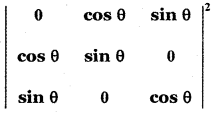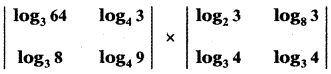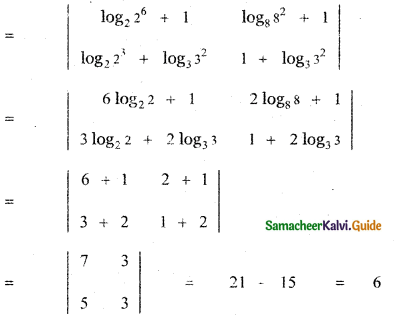Tamilnadu State Board New Syllabus Samacheer Kalvi 11th Maths Guide Pdf Chapter 7 Matrices and Determinants Ex 7.4 Text Book Back Questions and Answers, Notes.

## Tamilnadu Samacheer Kalvi 11th Maths Solutions Chapter 7 Matrices and Determinants Ex 7.4

Question 1.
Find the area of the triangle whose vertices are (0, 0), (1, 2) and (4, 3)
The given points are (0, 0), (1, 2) and (4, 3)
Area of the triangle with vertices
(x1, y1), (x2, y2) and (x3, y3) is∴ The area of the triangle with vertices
(0, 0), (1, 2) and (4, 3) is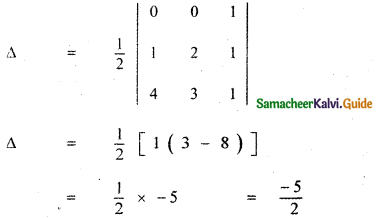Area cannot be negative. Taking positive value, we have
Required area Δ = $$\frac{5}{2}$$ sq.units.Question 2.
If (k, 2), (2, 4) and (3, 2) are vertices of the triangle of area 4 square units then determine the value of k.
Given Area of the triangle with vertices (k, 2), (2, 4) and (3, 2) is 4 square units.
The area of the triangle with vertices
(x1, y1) , (x2, y2) and (x3, y3) isGiven Δ = 4, (x1, y1) = (k , 2), (x2, y2) = (2 , 4) and (x3, y3) = (3 , 2)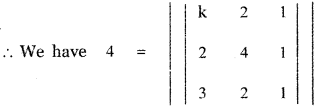± 4 = k(4 – 2) – 2 (2 – 3) + 1(4 – 12)
± 4 = k × 2 – 2 × – 1 – 8
± 4 = 2k + 2 – 8
± 4 = 2k – 6
2k – 6 = 4 or 2k – 6 = -4
2k = 4 + 6 or 2k = – 4 + 6
2k = 10 or 2k = 2
k = 5 or k = 1
Required values of k are 1, 5.Question 3.
Identify the singular and non – singular matrices.
(i) $$\left[ \begin{matrix} 1 & 2 & 3 \\ 4 & 5 & 6 \\ 7 & 8 & 9 \end{matrix} \right]$$
(ii) $$\left[ \begin{matrix} 2 & -3 & 5 \\ 6 & 0 & 4 \\ 1 & 5 & -7 \end{matrix} \right]$$
(iii) $$\left[ \begin{matrix} 0 & a\quad -\quad b & k \\ b-\quad a & 0 & 5 \\ -k & -5 & 0 \end{matrix} \right]$$
(i) $$\left[ \begin{matrix} 1 & 2 & 3 \\ 4 & 5 & 6 \\ 7 & 8 & 9 \end{matrix} \right]$$|A| = 1 (45 – 48) – 2(36 – 42) + 3(32 – 35)
|Al = – 3 – 2 × – 6 + 3 × – 3
|A| = – 3 + 12 – 9
|A| = – 12 + 12 = 0
∴ A is a singular matrix.(ii) $$\left[ \begin{matrix} 2 & -3 & 5 \\ 6 & 0 & 4 \\ 1 & 5 & -7 \end{matrix} \right]$$|B| = 2(0 – 20) + 3 (- 42 – 4) + 5(30 – 0)
|B| = -40 + 3 × – 46 + 150
|B| = -40 – 138 + 150
|B| = -178 + 150 ≠ 0
∴ B is non singular.

(iii) $$\left[ \begin{matrix} 0 & a\quad -\quad b & k \\ b-\quad a & 0 & 5 \\ -k & -5 & 0 \end{matrix} \right]$$|C| = 0 – (a – b) (0 + 5k) + k(-5 (b – a) – 0)
|C| = -5k (a – b) – 5k (b – a)
|C| = -5k (a – b) + 5k(a – b)
|C| = o
∴ C is a singular matrix.Question 4.
Determine the values of a and b so that the following matrices are singular:
(i) A = $$\left[ \begin{matrix} 7 & 3 \\ -2 & a \end{matrix} \right]$$
(ii) B = $$\left[ \begin{matrix} b\quad -\quad 1 & 2 & 3 \\ 3 & 1 & 2 \\ 1 & -2 & 4 \end{matrix} \right]$$
(i) A = $$\left[ \begin{matrix} 7 & 3 \\ -2 & a \end{matrix} \right]$$
|A| = $$\left[ \begin{matrix} 7 & 3 \\ -2 & a \end{matrix} \right]$$
|A| = 7a + 6
Given that A is singular
∴ |A| = 0
7a + 6 = 0 ⇒ a = $$\frac{-6}{7}$$

(ii) B = $$\left[ \begin{matrix} b\quad -\quad 1 & 2 & 3 \\ 3 & 1 & 2 \\ 1 & -2 & 4 \end{matrix} \right]$$
|B| = $$\left[ \begin{matrix} b\quad -\quad 1 & 2 & 3 \\ 3 & 1 & 2 \\ 1 & -2 & 4 \end{matrix} \right]$$
= (b – 1 )(4 + 4) – 2(12 – 2) + 3(- 6 – 1)
= 8 (b – 1) – 20 – 21
= 8b – 8 – 41
|B| = 8b -49
Given that B is singular
∴ |B| = 0
8b – 49 = 0 ⇒ b = $$\frac{49}{8}$$Question 5.
If cos 2θ = 0, determine